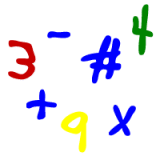Parents and Teachers: Support Ducksters by following us onor.

Kids Math

RatiosA ratio is a way to show a relationship or compare two numbers of the same kind.

We use ratios to compare things of the same type. For example, we may use a ratio to compare the number of boys to the number of girls in your class room. Another example would be to compare the number of peanuts to the number of total nuts in a jar of mixed nuts.

There are different ways we use to write ratios, and they all mean the same thing. Here are some of the ways you can write the ratios for the numbers of B (Boys) and G (Girls):

the ratio of B to G
B is to G
B:G

Note that when writing the ratio you place the first term first. This seems obvious, but when you see the question or ratio written as "the ratio of B to G" then you write the ratio B:G. If the ratio was written "the ratio of G to B" then you would write it as G:B.

Ratio Terminology

In the example above, B and G are terms. B is called the antecedent term and G is called the consequent term.

Example problem:

In a classroom with 15 total kids there are 3 kids with blue eyes, 8 kids with brown eyes, and 4 kids with green eyes. Find the following:

The ratio of blue eyed kids to kids in the class?

The number of blue eyed kids is 3. The number of kids is 15.
Ratio: 3:15

The ratio of brown eyed kids to green eyed kids?

The number of brown eyed kids is 8. the number of green eyed kids is 4.
Ratio: 8:4

Absolute values and reducing ratios

In the examples above we used the absolute values. In both cases these values could have been reduced. Just like with fractions, ratios can be reduced to their simplest form. We'll reduce the above ratios to their simplest form to give you an idea as to what this means. If you know how to reduce fractions, then you can reduce ratios.

The first ratio was 3:15. This can also be written as the fraction 3/15. Since 3 x 5 =15, this can be reduced, like a fraction, to 1:5. This ratio is the same as 3:15.

The second ratio was 8:4. This can be written as the fraction 8/4. This can be reduced all the way to 2:1. Again, this is the same ratio, but is reduced so that it is easier to understand.

For more on ratios see Ratios: Fractions, and Percentages

More Algebra Subjects
Algebra glossary
Exponents
Linear Equations - Introduction
Linear Equations - Slope Forms
Order of Operations
Ratios
Ratios, Fractions, and Percentages
Solving Algebra Equations with Addition and Subtraction
Solving Algebra Equations with Multiplication and Division

Back to Kids Math

Back to Kids StudyFollow us onor